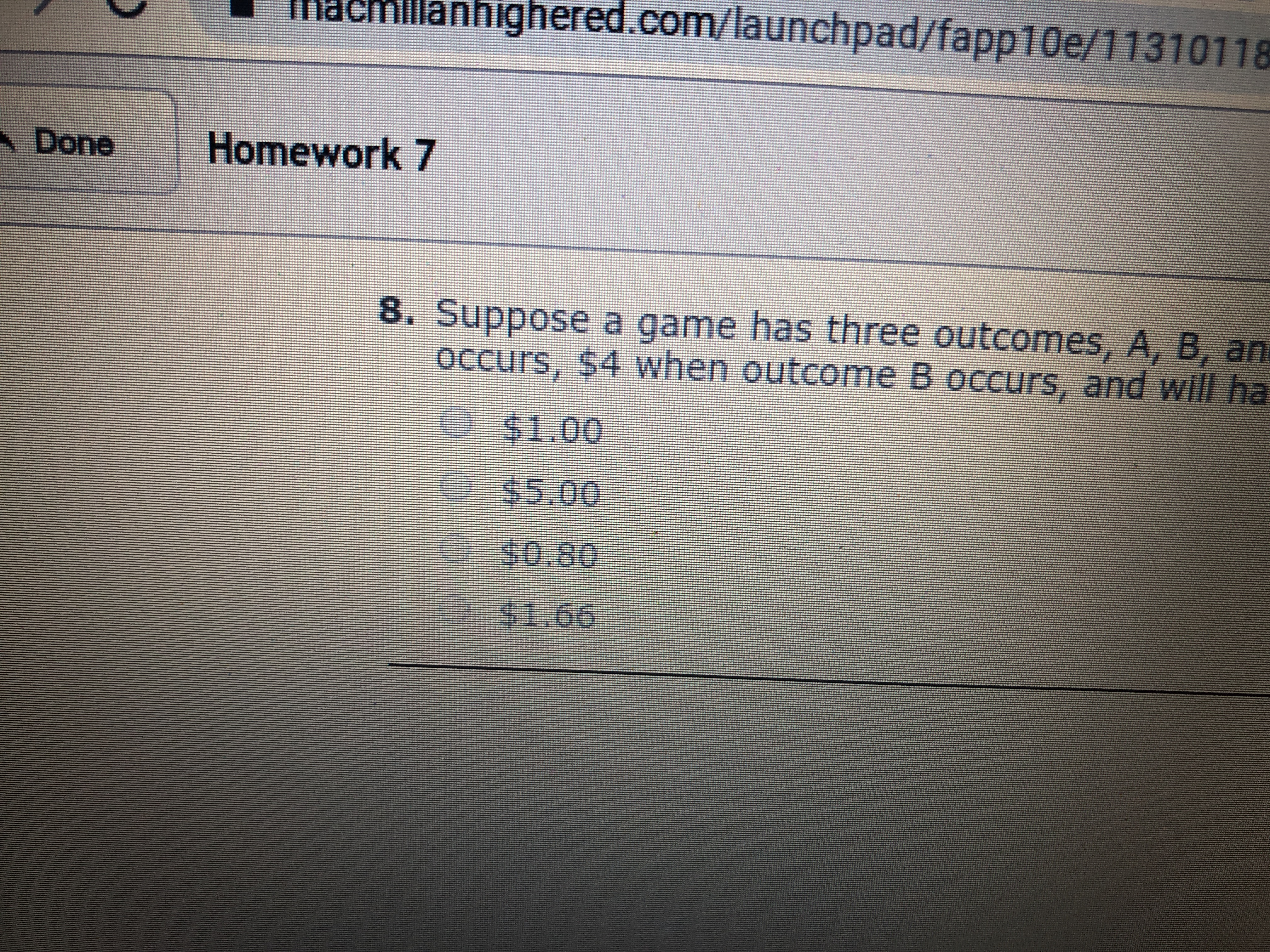# nhighered.com/launchpad/fapp10e/11310118 Iniach Done Homework 8. Suppose a game has three outcomes, A, B, an OCcurs, $4 when outcome B occurs, and will ha$1.00 $5.00 50.00$1.66

Question

Suppose a game has three outcomes, A, B and C with probabilities (A)=.2, (B)=.3, and (C)=.5.  A player will receive three dollars when outcome A occurs, four dollars when outcome B occurs, and will have to pay two dollars when outcome C occurs.  What is the mean value of one trial of the game?help_outlineImage Transcriptionclosenhighered.com/launchpad/fapp10e/11310118 Iniach Done Homework 8. Suppose a game has three outcomes, A, B, an OCcurs, $4 when outcome B occurs, and will ha$1.00 $5.00 50.00$1.66 fullscreen

### Want to see this answer and more?

Experts are waiting 24/7 to provide step-by-step solutions in as fast as 30 minutes!*

*Response times vary by subject and question complexity. Median response time is 34 minutes and may be longer for new subjects.
Tagged in
MathProbability

### Other